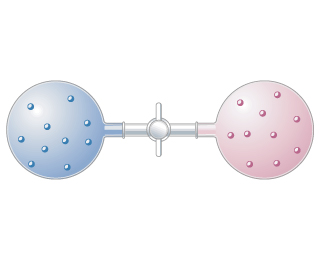# Problem: Two different gases occupy two separate bulbs. Consider the process that occurs when the stopcock separating the gases is opened, assuming the gases behave ideally.How does the process affect the enthalpy of the surroundings?

###### FREE Expert Solution

In this problem, we are asked about how the enthalpy of the surroundings is affected when the two gases are mixed together.

Enthalpy is a thermodynamic property that is defined as:

$\overline{){\mathbit{H}}{\mathbit{=}}{\mathbit{U}}{\mathbit{+}}{\mathbit{PV}}}$

Where is enthalpy, is internal energy, is pressure, and is volume.

And the change in enthalpy (ΔH) is equal to the heat that flows in and out of the system, in which, the enthalpy change of the universe is the sum of the enthalpy change of the system and the surroundings.

$\overline{){\mathbit{𝞓}}{{\mathbit{H}}}_{\mathbit{u}\mathbit{n}\mathbit{i}\mathbit{v}\mathbit{e}\mathbit{r}\mathbit{s}\mathbit{e}}{\mathbit{=}}{\mathbit{𝞓}}{{\mathbit{H}}}_{\mathbit{s}\mathbit{y}\mathbit{s}\mathbit{t}\mathbit{e}\mathbit{m}}{\mathbit{+}}{\mathbit{𝞓}}{{\mathbit{H}}}_{\mathbit{s}\mathbit{u}\mathbit{r}\mathbit{r}\mathbit{o}\mathbit{u}\mathbit{n}\mathbit{d}\mathbit{i}\mathbit{n}\mathbit{g}}}$

The system is the region in space that is subject to thermodynamic study. While the surrounding is everything that lies around the system.

To solve this problem, remember that:

ΔHsurr > 0: ΔHsystem < 0, the reaction is exothermic, heat is absorbed by the surrounding, heat is released by the system.

ΔHsurr < 0: ΔHsystem > 0, the reaction is endothermic, heat is absorbed by the system from the surrounding.

ΔHsurr = 0: ΔHsystem = 0, the reaction is neither endothermic nor exothermic, heat is neither lost nor gained.

99% (56 ratings)###### Problem Details

Two different gases occupy two separate bulbs. Consider the process that occurs when the stopcock separating the gases is opened, assuming the gases behave ideally.

How does the process affect the enthalpy of the surroundings?